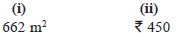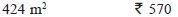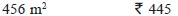# User Forum

Subject :IMO    Class : Class 6

(i) If the given figure (not drawn to scale) shows a rectangular field and shaded region shows the path of equal width, then find the area of the shaded region in the given figure.(ii) The cost of ploughing a field at ₹ 9 per square metre is ₹ 4104. If the field is 28.5 m long, then find the cost of fencing it at ₹ 5 per metre.

ABCD